Courses

# Machine Design - 1

## 15 Questions MCQ Test GATE Mechanical (ME) 2022 Mock Test Series | Machine Design - 1

Description
This mock test of Machine Design - 1 for GATE helps you for every GATE entrance exam. This contains 15 Multiple Choice Questions for GATE Machine Design - 1 (mcq) to study with solutions a complete question bank. The solved questions answers in this Machine Design - 1 quiz give you a good mix of easy questions and tough questions. GATE students definitely take this Machine Design - 1 exercise for a better result in the exam. You can find other Machine Design - 1 extra questions, long questions & short questions for GATE on EduRev as well by searching above.
QUESTION: 1

### An Aluminium rod of 30 mm diameter has a tensile load of 30 N on both ends of the rod. The yield and ultimate strengths of the Aluminium rod is 296 MPa and 379 MPa respectively. Determine the factor of safely

Solution: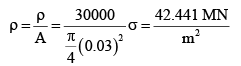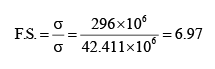QUESTION: 2

### Two plates are fixed together by means of rivets. Both rivets and plates are made of plain carbon steel 10C4 with yield strength of 250 MPa. The load of 5 kN is applied on both ends of the plates and the factor of safety is 2.5. Determine shear stress according to the principle of shear stress theory

Solution:

According to shear stress theory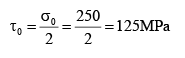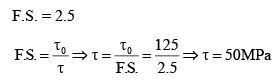QUESTION: 3

### The normal stresses and shear stresses are applied on the body are σx= 250 MPa, σ y= 180 MPa, and τxy= 80 MPa, respectively. Determine the normal stress at an oblique plane-making angle 60° with the horizontal axis.

Solution: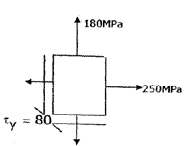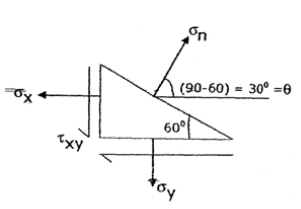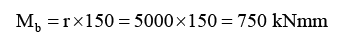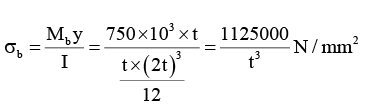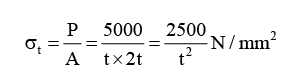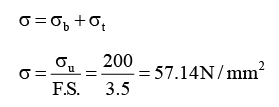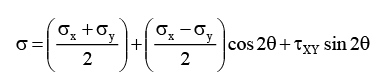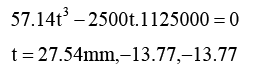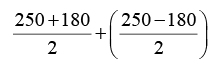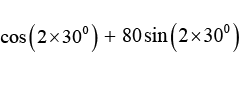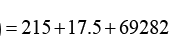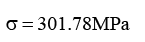QUESTION: 4

A flat plate 30mm wide and “t”mm wide is subjected to a tensile force of 5kN. The plate has a circular hole of diameter 15mm with the centre coinciding with the diagonal intersection point of the rectangle. If stress concentration factor=2.16, find the thickness of the plate if maximum allowable tensile stress is 80N/mm².

Solution:

σ=P/ (w-d) x t

σ=5000/(30-15)xt ;

σ(max)=K x σ

σ(max)=2.16 x σ

σ(max)=720/t;

Also, σ(max)=80.

so 80 = 720/t

t = 9 mm

QUESTION: 5

A 35 mm diameter beam is subjected to a reversed bending whose ultimate strength of the material is 450 MPa. Determine the endurance strength, take K a = 0.82, Kb = 0.85

Solution: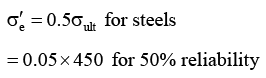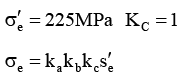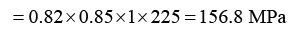QUESTION: 6

Two circular plates are clamped together by means of a bolt and nut with washer and the diameter of the bolt is 50 mm. The young’s modulus of the bolt and plates are 207 GPa and 71 GPa respectively. The initial preload in the bolt is 5 kN and the total length of the bolt is 80 mm. Determine the wrench torque required to create the preload

Solution: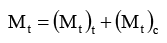(Mt) t =Torque required to overcome thread friction & reduce the preload

(Mt)1 =t orque required to overcome collar fiction between the nut and the washer.

Mt = 0.098P i d + 0.105P 1 d = 0.203P 1 d = 0.203×5000× 0.05

Mt = 5.075N-m

QUESTION: 7

For the diagram shown below, determine the thickness of weld whose permissible shear stress is 100 MPa.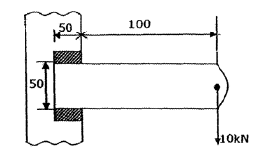Solution: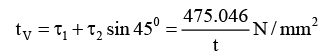Centre of gravity ‘a’ is located at ‘e’ = 25 + 100 = 125mm

Moment couple (M) = p × e = 10000× 125 = 1250000Nmm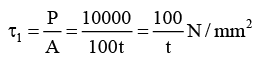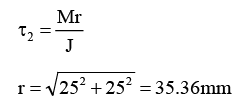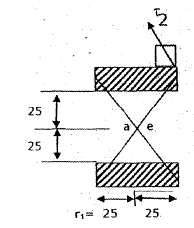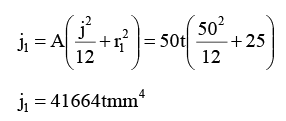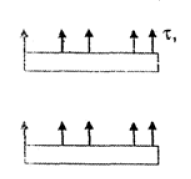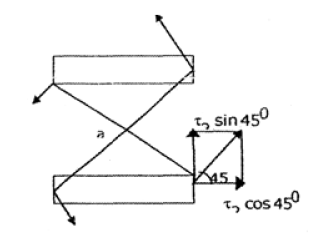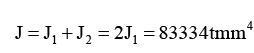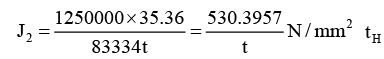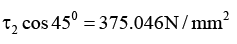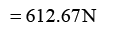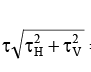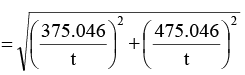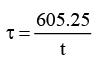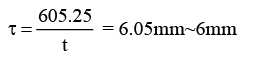QUESTION: 8

Two plates subjected to a tensile force of 50 kN are fixed together by means of two rivets perpendicular to the direction of the applied load. The tensile yield strength of the plates is 250 MPa. Factor of safety is 2.5. Neglect the stress concentration. The diameter of the rivet using the shear stress theory is ________ mm.

Solution: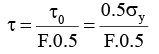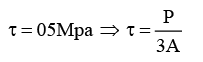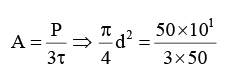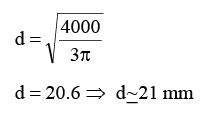QUESTION: 9

Match the following

List-I

(a) Efficiency of riveted joint

(b) Solid Bushing

(c) Ball Bearing

(d) In Bolt thread designation “N” stands for, example M36 is the designation

List-II

1. Maximum

2. Ration of least safe load to ultimate tensile strength of imperforated section

3. Metric

4. Rolling bearing

5. Sliding bearing

6. Ratio of highest safe load to ultimate tensile strength of imperforated section

Solution:
QUESTION: 10

The assemble of two circular plates, clamped together by a bolt whose length is 50 mm, outside diameter is twice the inner diameter and is subjected to a variable load varying from 0 to 10 kN. The ultimate and yield strength of the bolt material is 630 MPa and 380 MPa respectively. The Young’s modulus of bolt and plates material is 207 GPa & 71 GPa respectively. The surface finish factor is 0.75, size factor is 0.85 m reliability factor is 0.897, and the fatigue stress concentration factor is 2.2, the initial preload in the bolt is 3 kN.

Q.

Determine the endurance stress of the bolt material, (Assume steel)

Solution: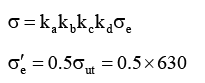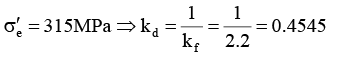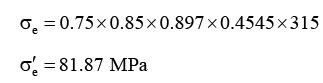QUESTION: 11

The assemble of two circular plates, clamped together by a bolt whose length is 50 mm, outside diameter is twice the inner diameter and is subjected to a variable load varying from 0 to 10 kN. The ultimate and yield strength of the bolt material is 630 MPa and 380 MPa respectively. The Young’s modulus of bolt and plates material is 207 GPa & 71 GPa respectively. The surface finish factor is 0.75, size factor is 0.85 m reliability factor is 0.897, and the fatigue stress concentration factor is 2.2, the initial preload in the bolt is 3 kN.

Q.

Determine the mean load on the bolt.

Solution: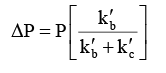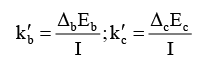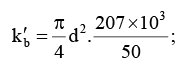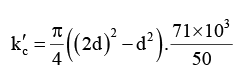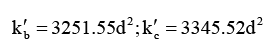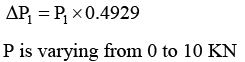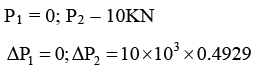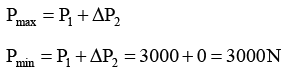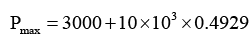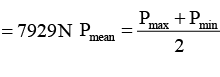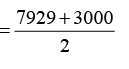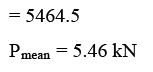QUESTION: 12

Determine the activating force to be applied for a single block brake with a torque capacity of 250 N-m. The drum rotates at 100 rpm and coefficient of friction is 0.35.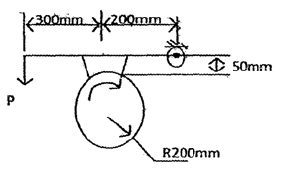Solution:

Free body diagram of lever is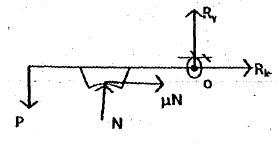QUESTION: 13

The gear train transmits a power of 3.5 kW at 750 rpm. The diameters of the two gears A and B are 300 mm and 600 mm respectively. The maximum tangential force at gear ‘A’ is __________ N.

(Take the service factor as 1.25)

Solution:
QUESTION: 14

Match List-I with List-II and select the correct answer using the codes given below the lists

List – I

(a) Activating force on the brake is zero

(b) Design of new friction lining

(c) Friction force helps to reduce the magnitude of activating force

(d) Addendum

List – II

1. Uncontrolled braking

2. Self-Energizing brake

3. Uniform pressure theory

4. Partially self-energizing brake

5. Uniform Wear theory

6. One module

Solution:

Activating force on the brake is zero is called Self-Energizing brake.

For a new friction lining, uniform pressure theory is used.

The friction force helps to reduce the magnitude of the actuating force P and a brake is called a partially self-energizing brake.

Addendum of gear is generally taken equal to 1 module.

QUESTION: 15

For the double long shoe, external drum brake illustrated in the figure (All dimensions are in mm), the face width of the shoes is 30 mm. the coefficient of friction between the shoe and the drum can be taken as 0.28.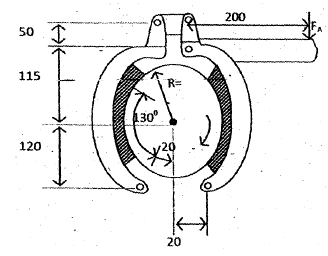Q.

Determine the actuation load on the left-had shoe when the pressure on the brake lining does not exceed 1.4 MPa

Solution: